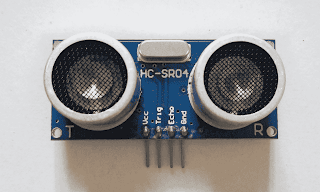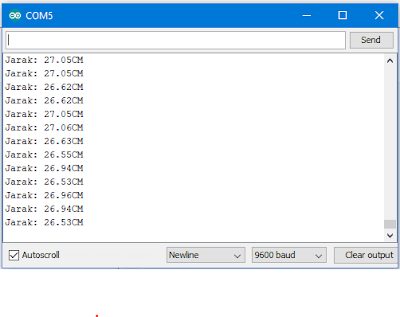# Ultrasonic Distance Sensor Arduino HC-SR04 Basic Program

## Ultrasonic Sensor working principle

Ultrasonic Distance Sensor Arduino HC-SR04 – Ultrasonic proximity sensors or often called PING sensors are sensors that work using high-frequency sound waves that can be used to determine the distance of an object.Contoh Sensor Jarak Arduino HC-SR04

Examples of applications of this sensor are determining the distance to park your car, measuring the height of people, knowing the presence of people at your door, and others.

The working principle is that the sensor generally has two funnels, the first one which is used for transmitting sound waves and the second which is used to receive the reflected waves.

The distance between the sensor and the object will be calculated using the formula:

Distance = speed of sound * T / 2

information:

• The speed of sound is 343 m/s.
• T is the travel time when the ultrasonic wave is transmitted and received again. “2” is the time it takes for sound waves to hit the object and bounce off the object.

### Basic Wiring Distance Sensor Ultrasonic

So that arduino can communicate with this hc-sr04 proximity sensor, then we must connect this sensor with arduino. follow the following wiring.

Now we will select the HC-SR04 sensor. This ultrasonic proximity sensor has 4 pins, namely Vcc, Gnd, Trig and Echo. Trig or Trigger pin is used for transmitte and Echo is used for receiving.

### Basic Program for Measuring Distance With Ultrasonic Sensor and Arduino

In the following, I provide a basic ultrasonic sensor coding on how Arduino can access the sensor and display distance data on the Arduino serial monitor.

Please connect the trigger pin from the ping sensor to pin 13 arduino and pin echo to pin 12. Then upload the following program.

```// -------------------------------------------
// HC-SR04 . Distance Detection Basic Program
// www.chippiko.com
// -------------------------------------------```
```#define pin_trig 13
#define pin_echo 12

void setup()
{
Serial.begin (9600);
pinMode(pin_trig, OUTPUT);
pinMode(pin_echo, INPUT);

}

void loop()
{
//send square wave
digitalWrite(pin_trig, HIGH);
delayMicroseconds(200);
digitalWrite(pin_trig, LOW);
delayMicroseconds(200);

double times = pulseIn(pin_echo, HIGH);

//calculate distance
double range = 0.0343 * ( times / 2);

Serial.print("Jarak: ");
Serial.print(range);
Serial.println("CM");
delay(100); // delay 100ms
}```

After the above program is uploaded to arduino, open the serial monitor and you will see the data display like this:Serial Monitor Sensor Ping
How Program works?

Trig pin will be High for 10 m/s then low.
digitalWrite(pin_trig, HIGH);
delayMicroseconds(10);
digitalWrite(pin_trig, LOW);

This sensor will transmit 40KHz square wave.

The reflected square wave will be received and will calculate how long it will take for this wave to be received.

In getting the time, we use the program line:

double times = pulseIn(pin_echo, HIGH);

Once the time is obtained, we can calculate the object distance using this formula “Distance = speed of sound * T/2”.

How to set this formula will measure in “cm”?

Since the speed of sound is 343 m/s or 34,300 cm/s, T uses m/s (1 m/s = 10-6), then:

Distance = 34,300 * (H / 10-6) / 2 = 0.0343 * (H / 2)

We use the program line:

double range = 0.0343 * ( times / 2);

This sr04 Arduino ultrasonic range sensor based on the datasheet is 4 meter max and  2 centimeter min.

### HC-SR04 Parking using Arduino

Now that we have the basic program for the Ping HC SR04 sensor, we will create another sample ultrasonic sensor arduino project using this sensor.

Connect your arduino to the HC-SR04 with the following pin configuration:

Arduino    HC-SR04
5V              Vcc
Gnd            Gnd
13              Trig
12             Echo
———————-
11 LED for notification in 10 cm
10 LED for notification in  25 cm
9 LED for notification in  40 cm
8 for Buzzer

We will make a car park. The working system is that the sensor will detect distances of 10cm, 25cm, and 40 cm.

When the sensor detects that distance, the buzzer will turn on.

The speed of the sound of the buzzer is proportional to the distance the sensor detects the object.

The closer the sensor detects the object, the faster the buzzer sounds.

Here is the program that we use on Arduino using the HC-SR04 Sensor:

```// -------------------------------------------
// sr04 arduino
// -------------------------------------------
#define pin_trig 13
#define pin_echo 12
#define pin_10cm 11
#define pin_25cm 10
#define pin_40cm 9
#define pin_buz 8

void setup()
{
//Serial.begin (9600)
pinMode(pin_trig, OUTPUT);
pinMode(pin_echo, INPUT);
pinMode(pin_10cm, OUTPUT);
pinMode(pin_25cm, OUTPUT);
pinMode(pin_40cm, OUTPUT);
pinMode(pin_buz, OUTPUT);
}

void loop()
{
//send wave square
digitalWrite(pin_trig, HIGH);
delayMicroseconds(10);
digitalWrite(pin_trig, LOW);

double times = pulseIn(pin_echo, HIGH);

//calculate of distance
double range = 0.0343 * ( times / 2);

//If the distance is approximately equal to 10cm
if (range <= 10 )
{
digitalWrite(pin_10cm, HIGH);
digitalWrite(pin_buz, HIGH);
Serial.print(range);
delay(50);
digitalWrite(pin_10cm, LOW);
digitalWrite(pin_buz, LOW);
delay(50);
}

//If the distance is more than 10.5 cm and less than 25 cm
if ((range >=10.5) && (range <= 25))
{
digitalWrite(pin_25cm, HIGH);
digitalWrite(pin_buz, HIGH);
Serial.println(range);
delay(100);
digitalWrite(pin_25cm, LOW);
digitalWrite(pin_buz, LOW);
delay(100);
}

//if the distance is more than 25.5 cm and less than 40 cm
if ((range >=25.5) && (range <= 40))
{
digitalWrite(pin_40cm, HIGH);
digitalWrite(pin_buz, HIGH);
Serial.println(range);
delay(200);
digitalWrite(pin_40cm, LOW);
digitalWrite(pin_buz, LOW);
delay(200);
}

else
{
digitalWrite(pin_10cm, LOW);
digitalWrite(pin_25cm, LOW);
digitalWrite(pin_40cm, LOW);
digitalWrite(pin_buz, LOW);
Serial.print(range);
Serial.println(" CM");
}```
`delay(100); // delay 100ms`
```}
```

The video of the code above is as follows:

Thus the program was created, hopefully the tutorial ultrasonic sensor arduino can be useful for you.

Thank you for visiting the Chip Piko website.

If you find this website useful, please share it using the following buttons: